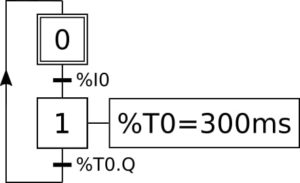# Timer – ST

The IEC 61131 standart offers a definition of Timers. PLC3000 integrates only TON timers that can be set by defining the time base %Ti.TB, and the preset value %Ti.PV. The timer is triggered by activating the input %Ti.IN, and the output %Ti.Q goes to 1 when the current timer value reaches the preset value.

The time bases %Ti.TB that can be declared are: 1, 10, 100 ms; 1, 10 s; 1 min.

Generation in ST

Let’s considering 3 timers with different time bases and preset values

```IF %S1 THEN
%T0.TB := 100ms;
%T1.TB := 1s;
%T2.TB := 1min;
%T0.PV := 3 ;
%T1.PV := 2;
%T2.PV := 1;
END_IF
```

Illustration with a Grafcet

Let’s considering the following GrafcetProgramming in ST – Step/Step with Crossing Bits

```PROGRAM StepStepBTimer (* TIMER *)
IF %S1 THEN
%T0.TB := 100ms;
%T0.PV := 3 ;
END_IF

(* STEP *)
%M10 := (%M1 AND %T0.Q) OR (%M0 AND NOT %I0) OR %S2;
%M11 := ((%M0 AND %I0) OR (%M1 AND NOT %T0.Q)) AND NOT %S2;

(* CROSSING *)
IF %M10 THEN
%M0 := TRUE;
%M1 := FALSE;
END_IF

IF %M11 THEN
%M1 := TRUE;
%M0 := FALSE;
END_IF

(* ACTIONS *)
%T0.IN := %M1;

END_PROGRAM
```